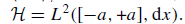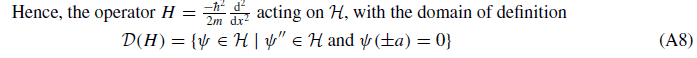# Is the kinetic energy of a particle quantized?

• B
Torog
Is the kinetic energy of a particle quantized? Does it increase and decrease in discreet amounts?

## Answers and Replies

Mentor
Is the kinetic energy of a particle quantized?

I think the answer to this question is "no", but the reason is that the question itself is based on an assumption: that kinetic energy is always an observable. I don't think that's true; I think kinetic energy is only an observable for the case of a free particle, for which the energy spectrum is continuous, not discrete, meaning kinetic energy is not quantized. The energy spectrum is discrete for the case of a particle in a bound system, but for such a particle, kinetic energy by itself is not an observable: only total energy is (i.e., including both kinetic and potential energy). So while total energy is quantized (has a discrete spectrum) for this case, you can't say that kinetic energy is, because you can't observe kinetic energy for this case.

•bhobba
Mentz114
Is the kinetic energy of a particle quantized? Does it increase and decrease in discreet amounts?
A charged particle in a magnetic field has quantized kinetic energy. It also has x and y velocity operators which do not commute with other nor their corresponding position operators. Ballentine treats this special case (where the particle is not free but has no potential energy) very readably.

•king vitamin
Gold Member
Firstly, the discreteness or continuity of an observable generally depends on the system in question. The total energy of some quantum systems, for example, is discrete, while in others is continuous, and in yet other systems is a mix of the two. So the question 'Is the such-and-such observable discrete or continuous' is not generally meaningful.

Secondly, if a system possesses any observable ##A##, then by the Spectral Theorem it also possesses any observable ##f(A)##, for any (sufficiently reasonable) function ##f##. So if a system has a valid momentum observable ##P##, then it also has a valid observable ##K = \frac {P^2} {2m}##, which is usually identified with kinetic energy.

Thirdly, the Spectral Mapping Theorem guarantees that the spectrum of ##f(A)## is related to the spectrum of ##A## by $$\sigma( f(A)) = \overline {f(\sigma (A))},$$ which means that in systems where the momentum is discrete, so too is the kinetic energy, and likewise when it is continuous.

•dextercioby
Torog
So as far as I can see the kinetic energy a particle could be continuous but we have no way of measuring it. We know it is quantized when it is in a system where we give it quantized pushes.

So we don't know much and there is no experiment we can do to understand my original question (thanks to Mr Heisenberg!). All we have is theory to guide us.

Mentor
The energy spectrum is discrete for the case of a particle in a bound system, but for such a particle, kinetic energy by itself is not an observable: only total energy is (i.e., including both kinetic and potential energy).
How about a particle in a square well? The boundary conditions lead to quantized total energies, but the potential is zero.

•Demystifier
Gold Member
How about a particle in a square well? The boundary conditions lead to quantized total energies, but the potential is zero.

Maybe there's some reason why the kinetic energy operator is not defined in a rigorous sense in that system (as is the case with the momentum operator). But you can get around that problem by considering a "particle on a ring" instead. Then there should be quantized kinetic energy eigenstates.

Homework Helper
Maybe there's some reason why the kinetic energy operator is not defined in a rigorous sense in that system (as is the case with the momentum operator). [...]

No, the KE operator is well-defined and self-adjoint on the following subset of#### Attachments

•hilbert2
Mentor
How about a particle in a square well? The boundary conditions lead to quantized total energies, but the potential is zero.

Hm, ok, yes, in this case the particle is bound but the total energy is the same as the kinetic energy.

I think that this question can only be answered: write the S equation of the particle under examination (there will be different cases if it is a free particle, rather than a particle in a potential square etc.) Finding the energy operator.
If the operator admits a continuous spectrum, the energy is continuous, if a discrete spectrum is admitted, only discrete values are admissible.

Mentor
Finding the energy operator.

Not just the "energy" operator--the kinetic energy operator, since that's specifically what the OP was asking about.